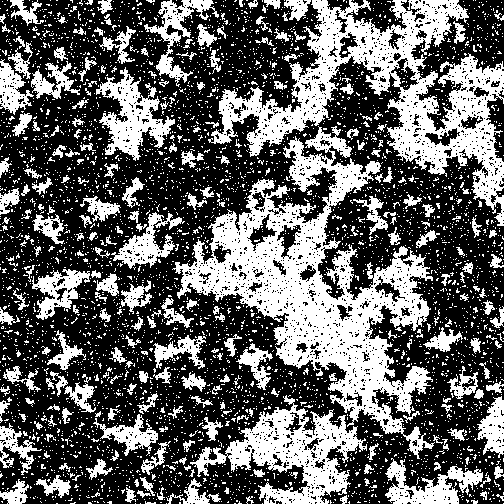# Split the differenceSuppose that the free energy of a system is described by $\mathcal{F}=(T-T_c)m^2+\frac14 m^4$ where $T$ is the temperature, and $m$ represents some property of the system, such as its overall magnetization. As $T$ rises and falls, but does not cross $T_c$, the allowed physical value(s) of $m$, $m^*$ change somewhat (see Assumptions).

When $\displaystyle T$ crosses $T_c$, however, there is a qualitative shift in the allowed values of $m^*$, and the system undergoes a phase transition.

Suppose we're working with the system at a temperature $\displaystyle T$ below $T_c$ such that $T-T_c=-10$, find $\lvert m^*\rvert$.

Assumptions

• The system always resides in states $m^*$ which minimize its free energy. These are the "physical" states of the system.
×